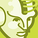# QlikView App Dev

Discussion Board for collaboration related to QlikView App Development.

Announcements
Our next Qlik Insider session will cover new key capabilities. Join us August 11th REGISTER TODAY
cancel
Showing results for
Did you mean:Not applicable

## what is difference between dimension and expression in qlikview ?

what is difference between dimension and expression in qlikview ?

1 Solution

Accepted SolutionsChampion

dimesion can be field  but expression could be sum(field),count(field) .you could calculate some amount .count some id

i mean you could apply functions in expression where in dimesion you could take direct fields

E:

i want to display sum of sales country wise

then in  dimesion i will take Country

and in expression i will write sum(sales)

hope this helps

10 RepliesChampion

dimesion can be field  but expression could be sum(field),count(field) .you could calculate some amount .count some id

i mean you could apply functions in expression where in dimesion you could take direct fields

E:

i want to display sum of sales country wise

then in  dimesion i will take Country

and in expression i will write sum(sales)

hope this helpsMVP

Hi Manoj,

To explain it in simple words:

Dimension acts as base data in the charts, on which all the calculation will get calculated.

Expression always works above the Dimension what you have used.

Eg.

Dimension

A

B

Expression sum(amount)......

in this case all the amount gets calculated on the dimension A and B.

Hope this helps u!!Not applicable
Author

Dimension is the set of values for the chart to iterate over when it calculates the values for its expression.

for eg. Year is dimension

and Expression which calculate values for dimension

for eg. Sales is expression

now the chart for above dimension and expression will show sales for Year.

Year     Sales

2009     150

2008     140

2010     147

the difference between them is

expression can be aggregated but dimension is not.

dimension can be grouped by Drill down but expression is not.Champion

Hi Bhawna,

we can also aggregate dimesions

for example

aggr(Max(date),EMPID)Not applicable
Author

Dimension - A dimension table is a table in a star schema of a data warehouse. A dimension table stores attributes, or dimensions, that describe the objects in a fact table.Expression- Expression is data script which is written to execute the function/formula.

Example:  " =Sum(Sales) " , "= Avg(Sales)"Not applicable
Author

thank youMaster III

Just study

"data warehouse concepts by ralph kimball"Not applicable
Author

thnX chauhan u gave me a simple example ..,nice i understandChampion

Country,Sales

India,100

US,200

Japan,300

];

take Country in dimension and sum(sales) in expressionCommunity Browser